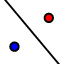# Reflections in the Coordinate Plane

1) Plot the points (type the ordered pair into the input bar, bottom of the page) 2) A(-3,2), B (-1,4) and C (-5, 3) 3)Use the polygon tool to construct the triangle ABC 4)Use the reflection tool (9th tool over, under the reflection diagram) 5) Select the Triangle ABC, then y-axis
When you're done (or if you're unsure of something), feel free to check by watching the quick silent screencast below the applet.

## Video (silent video)

What are the coordinates of A'B'C'?

## 2.

1) Plot the points (type the ordered pair into the input bar, bottom of the page) 2) A(5,-1), B (3,-1) and C (4, -3) 3)Use the polygon tool to construct the triangle ABC 4)Use the reflection tool (9th tool over, under the reflection diagram) 5) Select the Triangle ABC, then x-axis

List the points of A"B" and C"

If a Triangle is in quadrant 2, and we reflect it over the x_axis which quadrant would the image be in?